# AVL Trees in Data Structures

21 September, 2018

Ratings

## AVL Trees

One of the more popular balanced trees, known as an AVL tree in Data Structures, was introduced in 1962 by Adelson-Velski and Landis

#### Definition:

An empty binary search tree is an AVL tree. If T is a non empty binary search tree with T2 and TR as its left and right sub trees, The T is an AVL tree iff.

• T2 and TR are AVL trees and
• | hL – hR | < =1 where hL and hR are the heights of TL and T respectively

Or An Avl trees is a binary search tree in which for every node in the tree, The height of the left and right Sub trees differ by at most1.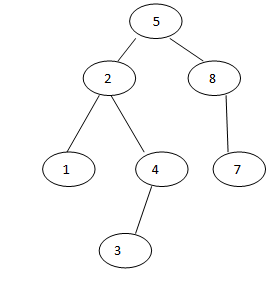hL – hR =1 This is AVL Tree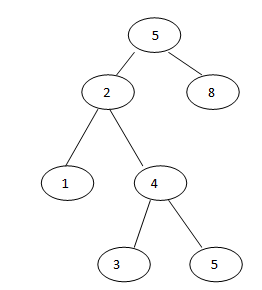hL – hR =2 This is not AVL Tree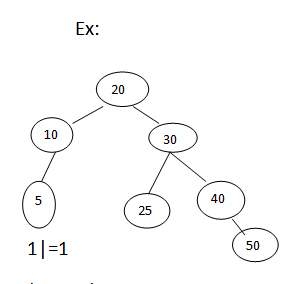This is also a AVL tree hL = Height of left sub tree =2 |hl – hR |=|2-3|=|- 1|=1 | hL –hR| <=1     The difference between heights at left sub tree and right sub tree is called balancing Factor of a node.   Importance of Rotations :

• The insert and delete operations of AVL tree are the same as binary search tree (BST)
• Since an insertion(deletion) involve adding (deleting) a tree node, this can only increase (decrease) the heights of same sub tree(s) by 1
• Thus, the AVL tree property may be violated
• If the AVL tree property is violated ata node x, it means that the height of left(x) and right(x) differ by exactly 2
• After the insertion or deletion operations, we need to examine the tree and see if any node violates the AVL tree property
• If the AVL tree property is violated at node so, single or double rotation will be applied to x to restore the AVL tree property.
• Rotation will be applied in a bottom up manner starting at the place of insertion(deletion)
• Thus when we perform a rotation at x, The AVL tree property is restored at all proper descendants of x.

This fact is important.   Single Rotations : RR rotation :- [ Right – Right Rotation]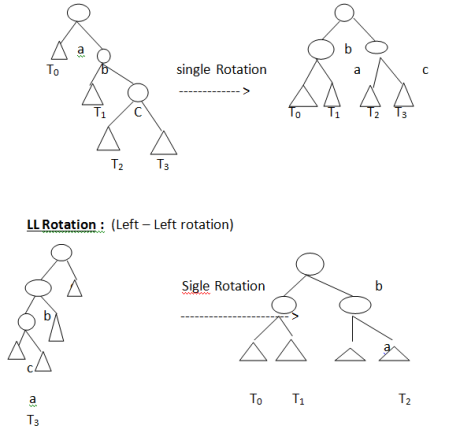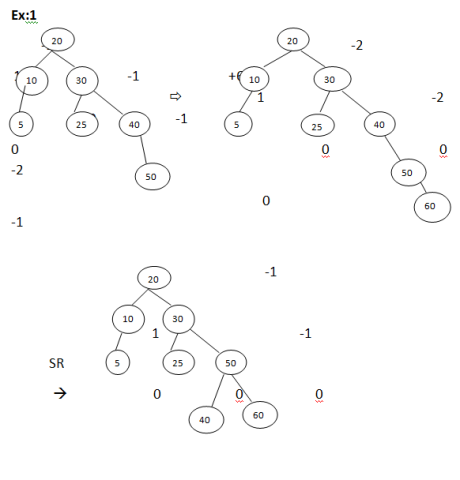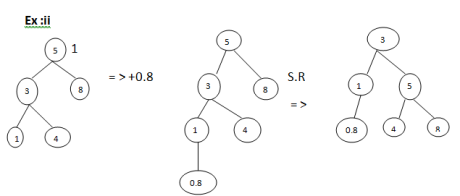### Double Rotations :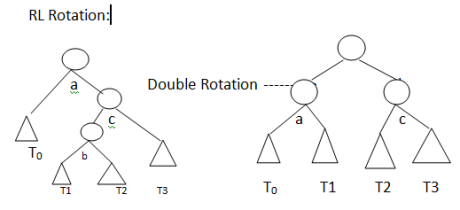### LR Rotaion  c Left Right Rotation :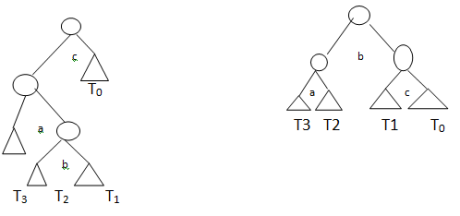Ex : Example of RL Rotation :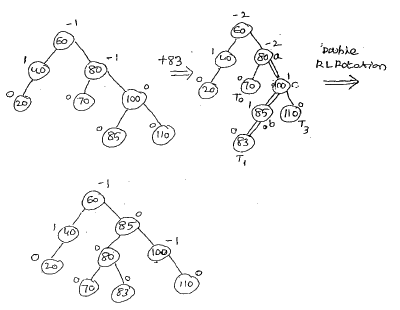### Ex: Example of LR Rotation:Example: Construct an AVL tree with the following elements 3,2,1,4,5,6,716,15,14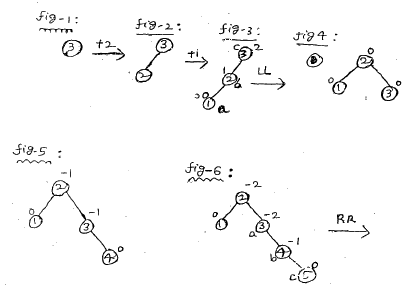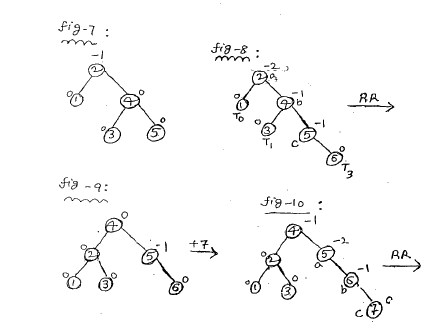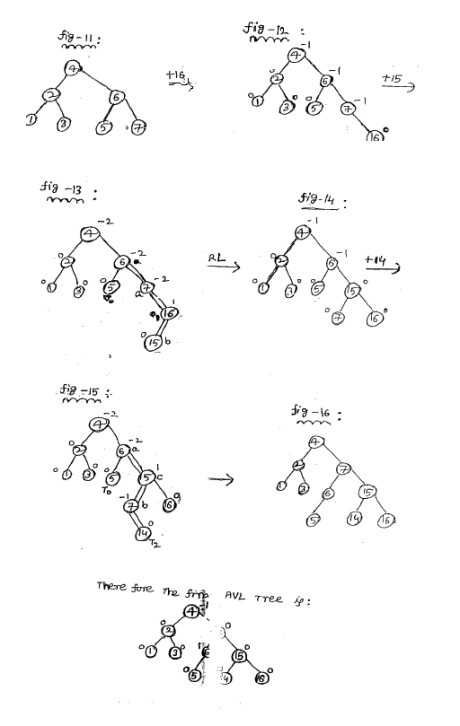### Insertion :

• Trace from path of inserted leaf towards the root, and check if the AVL tree property is violated perform rotation if necessary
• For insertion, once we perform (Single or doubles) rotation at a node x, The AVL tree property is already restored. We need not to perform any rotation at any ancestor of X.
• Thus one rotation is enough to restore the AVL tree properly
• There are 4 different cases (actually 2) , so don’t mix up them
• The time complexity to perform rotation is 0(1)
• The time completely to insert, and find a node that violates the AVL property is dependent on the height of the tree, which is 0(log(n))
• So insertion takes o(log(n))

### Deletion :

• Delete a node X as in ordinary BST(Note that X is either a leaf or X has exactly one child)
• Check and restore the AVL tree property.
• Trace from path of deleted node towards the root and check if the AVL tree property is violated
• Similar to an insertion operation, there are four cases to restore the AVL tree property.
• The only difference from insertion is that after we perform a rotation at x, we may have to perform, a rotation at some ancestors of x, It may involve several rotations.
• Therefore, we must continue to trace the path until we reach the root.
• The time complexity to delete a node is dependent on the height of the tree, which is also o(log(n))